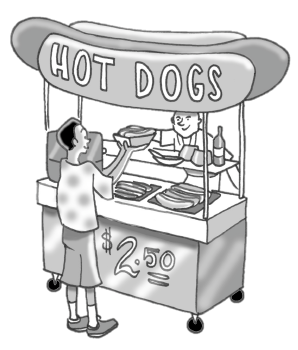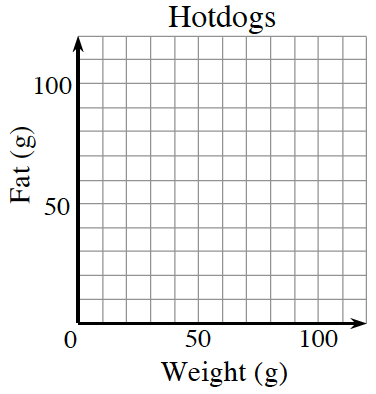Home > ACC7 > Chapter cc31 > Lesson cc31.1.2 > Problem1-13

1-13.

Copy the axes below onto your paper. Add an appropriate scale and then place and label a point on the graph for each of the products listed below. Homework Help ✎

Create ordered pairs for each hot dog before plotting.

Decide what information is your independent variable ($x$) and what information is your dependent variable ($y$).

1. Dog-Eat-Dog has a supreme hotdog that weighs $80$ grams and has $40$ grams of fat.

2. Hot Doggies has a diet hotdog that weighs $50$ grams and has only $9$ grams of fat.

3. Dog-alicious has a cheap hotdog that weighs $40$ grams and has $30$ grams of fat.